# NCERT solutions for Mathematics Exemplar Class 9 chapter 9 - Areas of Parallelograms & Triangles [Latest edition]

#### Chapters## Chapter 9: Areas of Parallelograms & Triangles

Exercise 9.1Exercise 9.2Exercise 9.3Exercise 9.4
Exercise 9.1 [Pages 85 - 87]

### NCERT solutions for Mathematics Exemplar Class 9 Chapter 9 Areas of Parallelograms & Triangles Exercise 9.1 [Pages 85 - 87]

#### Choose the correct alternative:

Exercise 9.1 | Q 1 | Page 85

The median of a triangle divides it into two ______.

• Triangles of equal area

• Congruent triangles

•  right triangles

• Isosceles triangles

Exercise 9.1 | Q 2 | Page 85

In which of the following figures, you find two polygons on the same base and between the same parallels?

•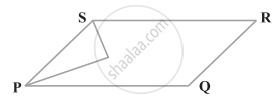•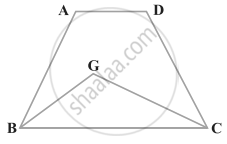•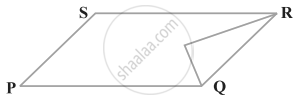•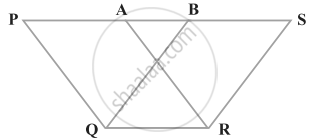Exercise 9.1 | Q 3 | Page 86

The figure obtained by joining the mid-points of the adjacent sides of a rectangle of sides 8 cm and 6 cm is ______.

• a rhombus of area 24 cm2

• a rectangle of area 24 cm2

• a square of area 26 cm2

• a trapezium of area 14 cm2

Exercise 9.1 | Q 4 | Page 86

In figure, the area of parallelogram ABCD is ______.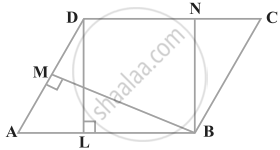• AB × BM

• BC × BN

• DC × DL

Exercise 9.1 | Q 5 | Page 86

In figure if parallelogram ABCD and rectangle ABEF are of equal area, then ______.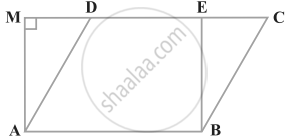• Perimeter of ABCD = Perimeter of ABEM

• Perimeter of ABCD < Perimeter of ABEM

• Perimeter of ABCD > Perimeter of ABEM

• Perimeter of ABCD = 1/2 (Perimeter of ABEM)

Exercise 9.1 | Q 6 | Page 87

The mid-points of the sides of a triangle ABC along with any of the vertices as the fourth point make a parallelogram of area equal to ______.

• 1/2 ar (ABC)

• 1/3 ar (ABC)
• 1/4 ar (ABC)
• ar (ABC)
Exercise 9.1 | Q 7 | Page 87

Two parallelograms are on equal bases and between the same parallels. The ratio of their areas is ______.

• 1:2

• 1:1

• 2:1

• 3:1

Exercise 9.1 | Q 8 | Page 87

ABCD is a quadrilateral whose diagonal AC divides it into two parts, equal in area, then ABCD ______.

• Is a rectangle

• Is always a rhombus

• Is a parallelogram

• Need not be any of (A), (B) or (C)

Exercise 9.1 | Q 9 | Page 87

If a triangle and a parallelogram are on the same base and between same parallels, then the ratio of the area of the triangle to the area of parallelogram is ______.

• 1:3

• 1:2

• 3:1

• 1:4

Exercise 9.1 | Q 10 | Page 87

ABCD is a trapezium with parallel sides AB = a cm and DC = b cm (figure). E and F are the mid-points of the non-parallel sides. The ratio of ar (ABFE) and ar (EFCD) is ______.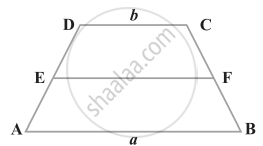• a : b

• (3a + b) : (a + 3b)

• (a + 3b) : (3a + b)

• (2a + b) : (3a + b)

Exercise 9.2 [Page 88]

### NCERT solutions for Mathematics Exemplar Class 9 Chapter 9 Areas of Parallelograms & Triangles Exercise 9.2 [Page 88]

#### State whether the following statement is True or False:

Exercise 9.2 | Q 1 | Page 88

ABCD is a parallelogram and X is the mid-point of AB. If ar (AXCD) = 24 cm2, then ar (ABC) = 24 cm2.

• True

• False

Exercise 9.2 | Q 2 | Page 88

PQRS is a rectangle inscribed in a quadrant of a circle of radius 13 cm. A is any point on PQ. If PS = 5 cm, then ar (PAS) = 30 cm2.

• True

• False

Exercise 9.2 | Q 3 | Page 88

PQRS is a parallelogram whose area is 180 cm2 and A is any point on the diagonal QS. The area of ∆ ASR = 90 cm2.

• True

• False

Exercise 9.2 | Q 4 | Page 88

ABC and BDE are two equilateral triangles such that D is the mid-point of BC. Then ar (BDE) = 1/4 ar (ABC).

• True

• False

Exercise 9.2 | Q 5 | Page 88

In figure, ABCD and EFGD are two parallelograms and G is the mid-point of CD. Then ar (DPC) = 1/2 ar(EFGD).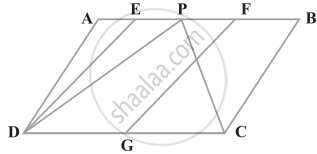• True

• False

Exercise 9.3 [Pages 89 - 92]

### NCERT solutions for Mathematics Exemplar Class 9 Chapter 9 Areas of Parallelograms & Triangles Exercise 9.3 [Pages 89 - 92]

Exercise 9.3 | Q 1 | Page 89

In figure, PSDA is a parallelogram. Points Q and R are taken on PS such that PQ = QR = RS and PA || QB || RC. Prove that ar (PQE) = ar (CFD).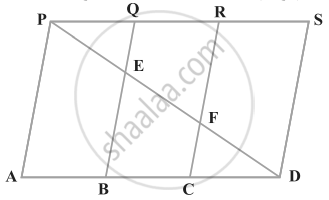Exercise 9.3 | Q 2 | Page 90

X and Y are points on the side LN of the triangle LMN such that LX = XY = YN. Through X, a line is drawn parallel to LM to meet MN at Z (See figure). Prove that ar (LZY) = ar (MZYX)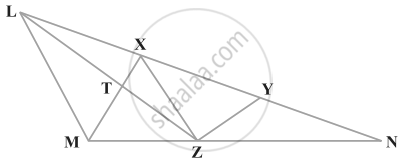Exercise 9.3 | Q 3.(i) | Page 90

The area of the parallelogram ABCD is 90 cm2 (see figure). Find ar (ΔBEF)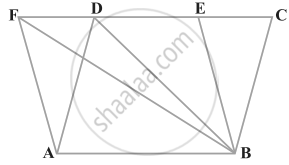Exercise 9.3 | Q 3.(ii) | Page 90

The area of the parallelogram ABCD is 90 cm2 (see figure). Find ar (ΔABD)Exercise 9.3 | Q 3.(iii) | Page 90

The area of the parallelogram ABCD is 90 cm2 (see figure). Find ar (ΔBEF)Exercise 9.3 | Q 4 | Page 90

In ∆ABC, D is the mid-point of AB and P is any point on BC. If CQ || PD meets AB in Q (figure), then prove that ar (BPQ) = 1/2 ar(∆ABC).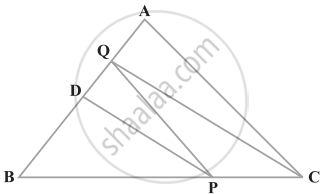Exercise 9.3 | Q 5 | Page 90

ABCD is a square. E and F are respectively the midpoints of BC and CD. If R is the mid-point of EF (figure), prove that ar (AER) = ar (AFR)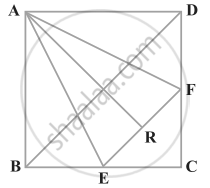Exercise 9.3 | Q 6 | Page 91

O is any point on the diagonal PR of a parallelogram PQRS (figure). Prove that ar (PSO) = ar (PQO).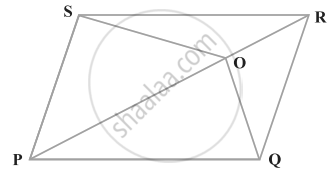Exercise 9.3 | Q 7 | Page 91

ABCD is a parallelogram in which BC is produced to E such that CE = BC (figure). AE intersects CD at F. If ar (DFB) = 3 cm2, find the area of the parallelogram ABCD.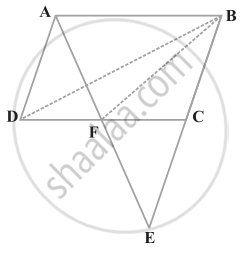Exercise 9.3 | Q 8 | Page 91

In trapezium ABCD, AB || DC and L is the mid-point of BC. Through L, a line PQ || AD has been drawn which meets AB in P and DC produced in Q (figure). Prove that ar (ABCD) = ar (APQD)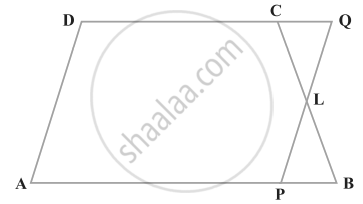Exercise 9.3 | Q 9 | Page 92

If the mid-points of the sides of a quadrilateral are joined in order, prove that the area of the parallelogram so formed will be half of the area of the given quadrilateral (figure).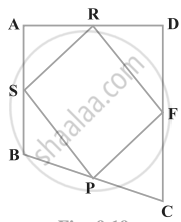Exercise 9.4 [Pages 94 - 96]

### NCERT solutions for Mathematics Exemplar Class 9 Chapter 9 Areas of Parallelograms & Triangles Exercise 9.4 [Pages 94 - 96]

Exercise 9.4 | Q 1 | Page 94

A point E is taken on the side BC of a parallelogram ABCD. AE and DC are produced to meet at F. Prove that ar (ADF) = ar (ABFC)

Exercise 9.4 | Q 2 | Page 94

The diagonals of a parallelogram ABCD intersect at a point O. Through O, a line is drawn to intersect AD at P and BC at Q. Show that PQ divides the parallelogram into two parts of equal area.

Exercise 9.4 | Q 3 | Page 94

The medians BE and CF of a triangle ABC intersect at G. Prove that the area of ∆GBC = area of the quadrilateral AFGE.

Exercise 9.4 | Q 4 | Page 95

In figure, CD || AE and CY || BA. Prove that ar (CBX) = ar (AXY).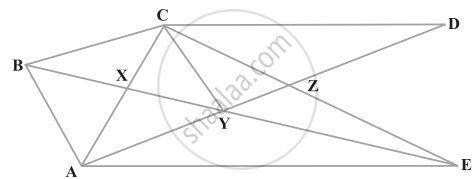Exercise 9.4 | Q 5 | Page 95

ABCD is a trapezium in which AB || DC, DC = 30 cm and AB = 50 cm. If X and Y are, respectively the mid-points of AD and BC, prove that ar (DCYX) = 7/9 ar (XYBA

Exercise 9.4 | Q 6 | Page 95

In ∆ABC, if L and M are the points on AB and AC, respectively such that LM || BC. Prove that ar (LOB) = ar (MOC)

Exercise 9.4 | Q 7 | Page 95

In figure, ABCDE is any pentagon. BP drawn parallel to AC meets DC produced at P and EQ drawn parallel to AD meets CD produced at Q. Prove that ar (ABCDE) = ar (APQ)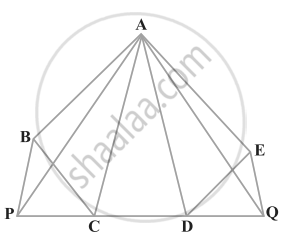Exercise 9.4 | Q 8 | Page 96

If the medians of a ∆ ABC intersect at G, show that ar (AGB) = ar (AGC) = ar (BGC) = 1/3 ar (ABC)

Exercise 9.4 | Q 9 | Page 96

In figure, X and Y are the mid-points of AC and AB respectively, QP || BC and CYQ and BXP are straight lines. Prove that ar (ABP) = ar (ACQ).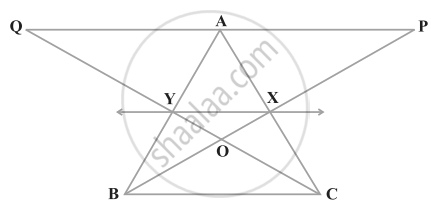Exercise 9.4 | Q 10 | Page 96

In figure, ABCD and AEFD are two parallelograms. Prove that ar (PEA) = ar (QFD).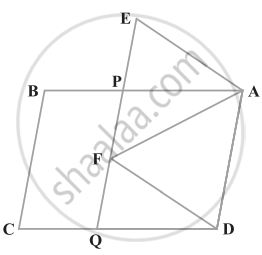## Chapter 9: Areas of Parallelograms & Triangles

Exercise 9.1Exercise 9.2Exercise 9.3Exercise 9.4## NCERT solutions for Mathematics Exemplar Class 9 chapter 9 - Areas of Parallelograms & Triangles

NCERT solutions for Mathematics Exemplar Class 9 chapter 9 (Areas of Parallelograms & Triangles) include all questions with solution and detail explanation. This will clear students doubts about any question and improve application skills while preparing for board exams. The detailed, step-by-step solutions will help you understand the concepts better and clear your confusions, if any. Shaalaa.com has the CBSE Mathematics Exemplar Class 9 solutions in a manner that help students grasp basic concepts better and faster.

Further, we at Shaalaa.com provide such solutions so that students can prepare for written exams. NCERT textbook solutions can be a core help for self-study and acts as a perfect self-help guidance for students.

Concepts covered in Mathematics Exemplar Class 9 chapter 9 Areas of Parallelograms & Triangles are Corollary: Triangles on the same base and between the same parallels are equal in area., Corollary: A rectangle and a parallelogram on the same base and between the same parallels are equal in area., Theorem: Parallelograms on the Same Base and Between the Same Parallels., Concept of Area.

Using NCERT Class 9 solutions Areas of Parallelograms & Triangles exercise by students are an easy way to prepare for the exams, as they involve solutions arranged chapter-wise also page wise. The questions involved in NCERT Solutions are important questions that can be asked in the final exam. Maximum students of CBSE Class 9 prefer NCERT Textbook Solutions to score more in exam.

Get the free view of chapter 9 Areas of Parallelograms & Triangles Class 9 extra questions for Mathematics Exemplar Class 9 and can use Shaalaa.com to keep it handy for your exam preparation